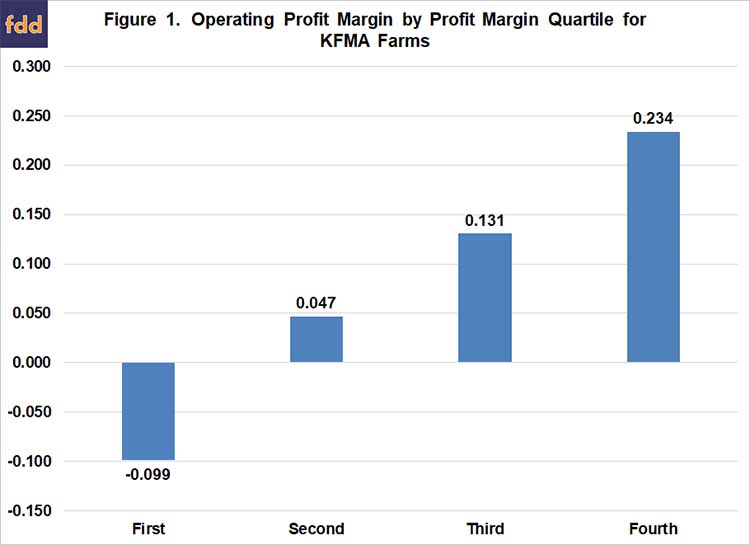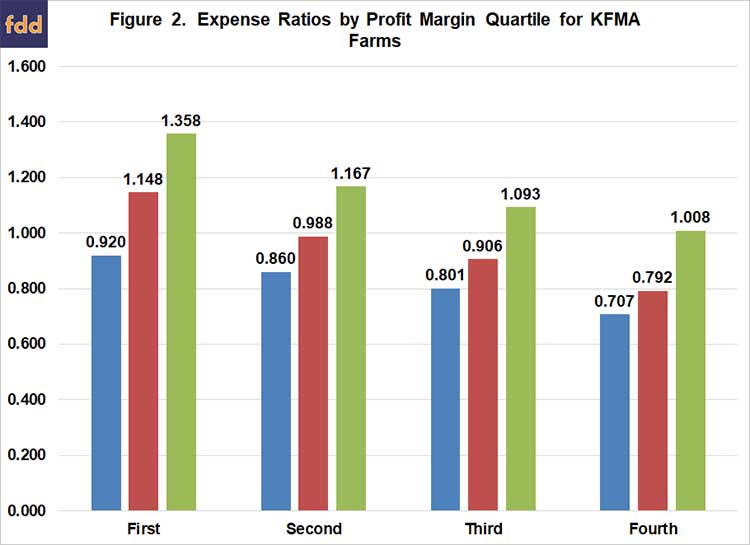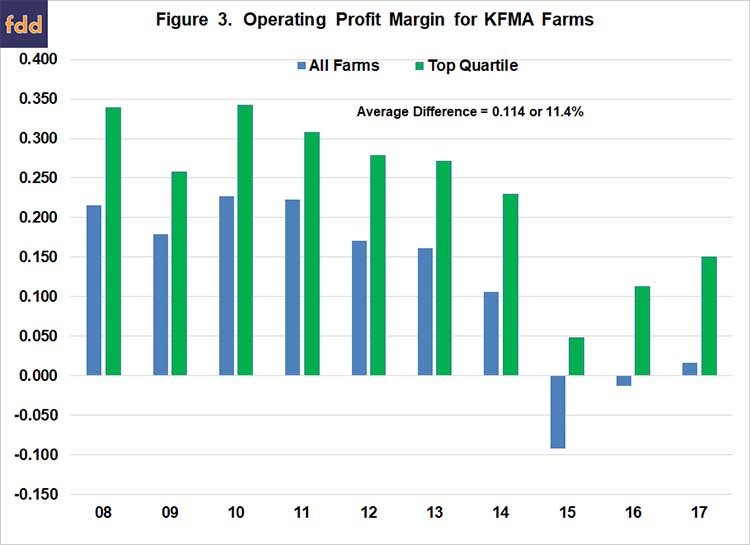# Operating Profit Margin Benchmarks

August 24, 2018
farmdoc daily (8):159
Recommended citation format: Langemeier, M. and E. Yeager. "Operating Profit Margin Benchmarks." farmdoc daily (8):159, Department of Agricultural and Consumer Economics, University of Illinois at Urbana-Champaign, August 24, 2018.

This article examines trends in the operating profit margin for a sample of farms over a ten-year period and develops financial performance benchmarks.  Specifically, using KFMA whole-farm data for farms with continuous data from 2008 to 2017, the operating profit margin ratio is computed for each farm and year.  In addition to developing a benchmark for the operating profit margin ratio, expense ratio benchmarks are discussed.

#### Benchmark Definitions

The operating profit margin ratio was computed by adding interest expense and subtracting unpaid family and operator labor from net farm income and dividing the result by the value of farm production.  In addition to the operating profit margin, expense ratios are compared across profit margin quartiles.  The total expense ratio was computed by summing cash costs, accrual adjustments to costs, and depreciation, and dividing the result by value of farm production.  The adjusted total expense ratio was computed by adding unpaid family and operator labor to the expenses included in the total expense ratio and dividing by value of farm production.  An adjusted total expense ratio below 1.00 indicates that a farm was able to cover accrual expenses, depreciation, and unpaid family and operator labor.  The economic total expense ratio was computed by adding the opportunity cost on net worth to the expenses in the adjusted total expense ratio and dividing by value of farm production.  If the economic total expense ratio was below 1.00, the farm or group of farms was covering all accrual and opportunity expenses, and was earning an economic profit.

#### Profit Margin Quartiles

Before discussing financial performance across profit margin quartiles, we will highlight averages for the sample of farms for the 2008 to 2017 period.  Value of farm production averaged \$592,485 and net farm income averaged \$121,487.  The average profit margin was 0.121 or 12.1 percent.  The average total expense ratio, adjusted total expense ratio, and economic total expense ratio were 0.795, 0.913, and 1.111, respectively.  Approximately 65 percent of the farms had an adjusted expense ratio below 1.0, and approximately 16 percent of the farms covered all accrual and opportunity costs and thus were earning an economic profit.

Figure 1 presents the operating profit margin ratio for each quartile.  This figure was created using ten-year average data for each farm.  The first quartile represents farms in the bottom quartile while the fourth quartile represents farms in the top quartile.  The farms in the top profit margin quartile had an average operating profit margin ratio of 0.234 or 23.4 percent.  In contrast, the farms in the bottom profit margin quartile had an average operating profit margin ratio of -0.099.The farms in the bottom profit margin quartile had relatively high expense ratios (Figure 2).  In fact, only 83 percent of the farms in the bottom profit margin quartile had a total expense ratio below 1.0, and none of these farms had an adjusted total expense ratio or economic total expense ratio below 1.0.  In contrast, all of the farms in top quartile covered accrual expenses, depreciation, and unpaid family and operator labor (i.e., had an adjusted total expense ratio below 1.0).  Moreover, approximately 41 percent of the farms in the top profit quartile earned an economic profit.Though not emphasized in this paper, we examined the percentage of farms in each size category that were included in each profit margin quartile.  The farms in the top profit margin quartile tended to be larger than the farms in the bottom quartile.  However, there were farms in each farm size category in the top quartile.

Figure 3 presents the average annual operating profit margin ratio for the entire sample of farms and for farms in the top quartile.  The average profit margin for the entire sample was negative in 2015 and 2016 and close to zero in 2017.  For farms in the top quartile, the average profit margin ranged from 5 percent in 2015 to 15 percent in 2017 for these same years.  Figure 3 also stresses the importance of using multiple years to benchmark farms.  For example, a 20 percent profit margin was relatively easy to attain in 2008, 2010, and 2011.  For 2015 to 2017, this benchmark would have been very difficult to achieve.